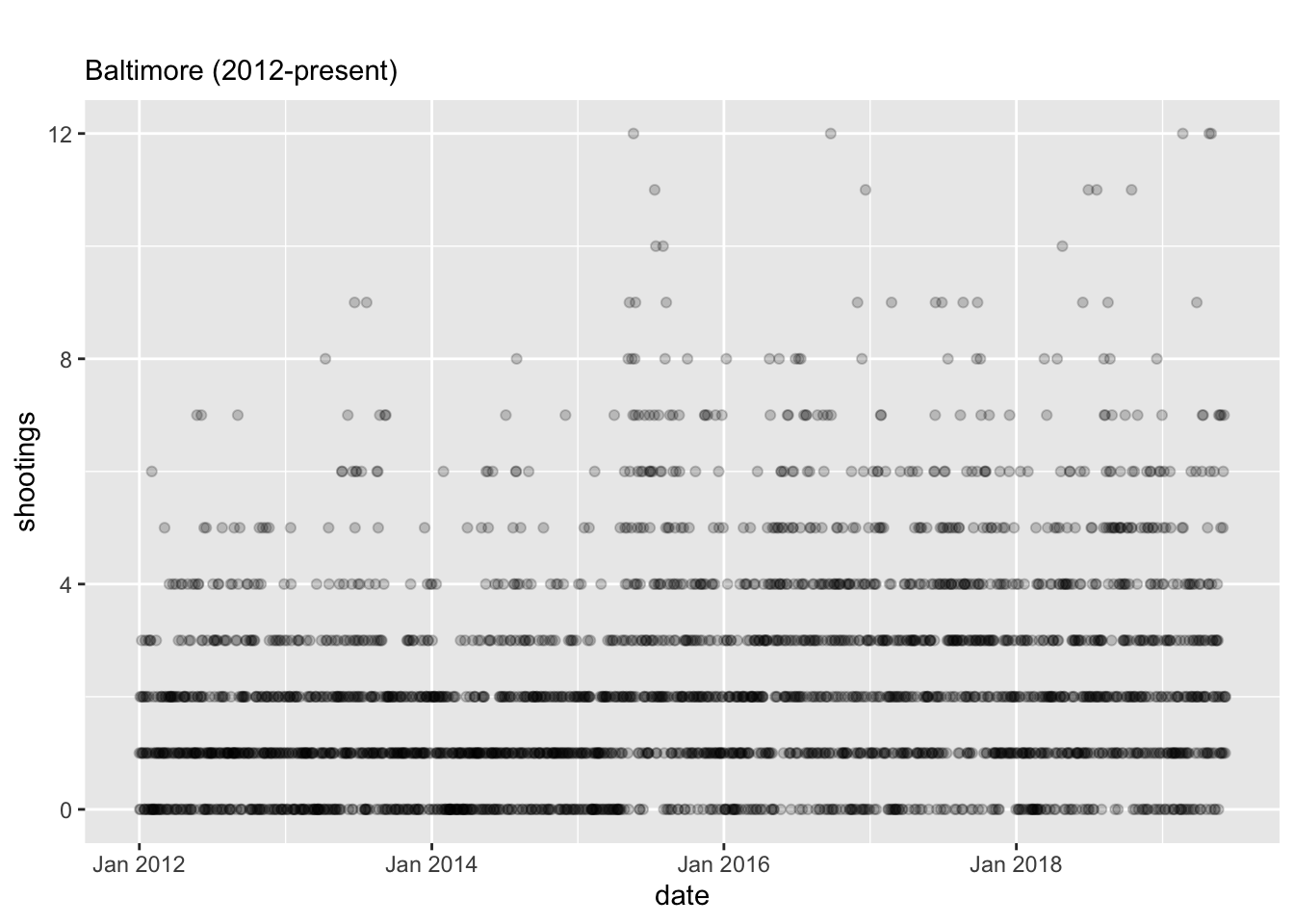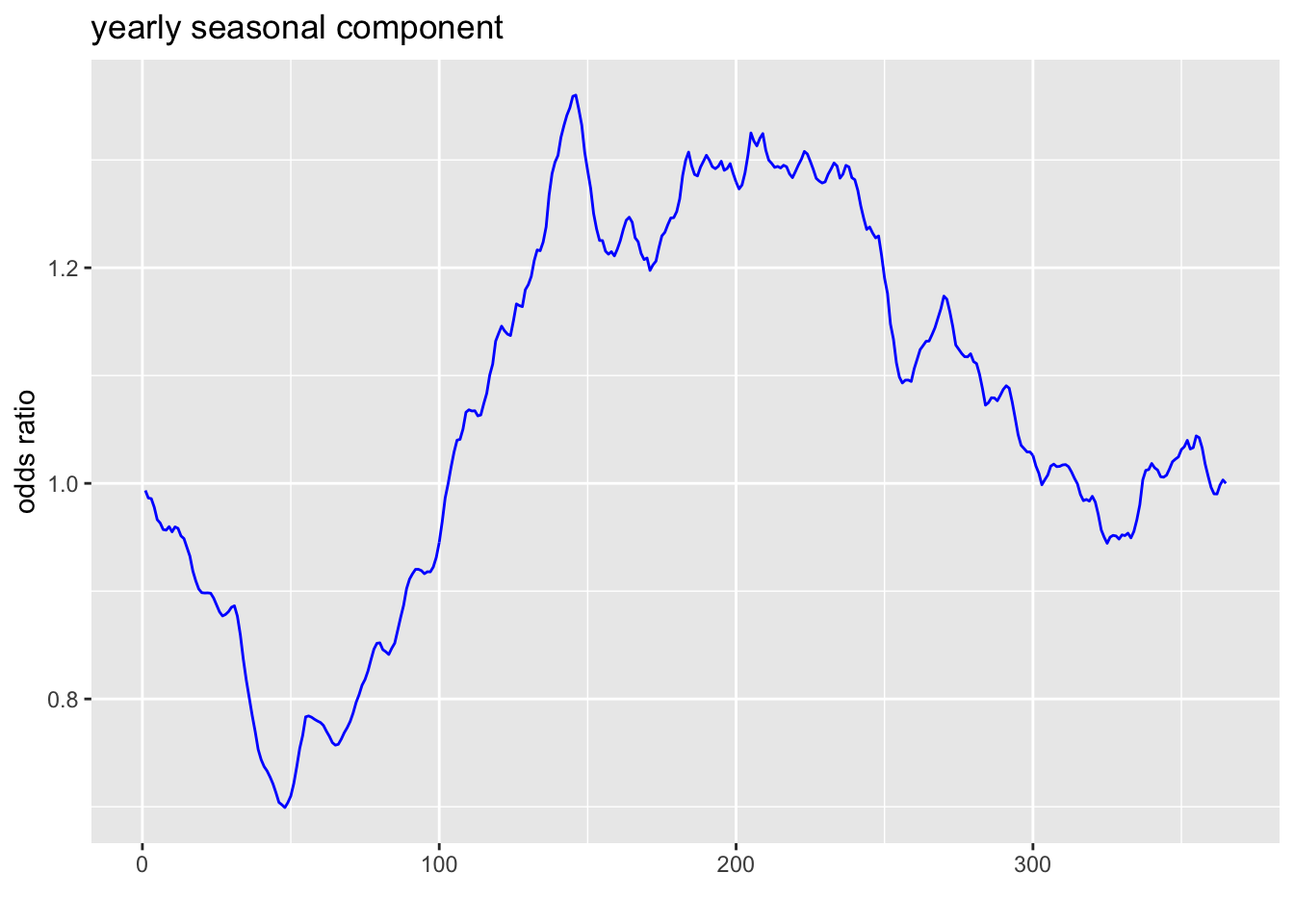In this markdown I had modeled shootings in Baltimore (from 2012-present) using a Bayesian generalized additive model. This has a lot of benefits mostly in terms of the ease of interpreting the model components and the ease of writing up the model (e.g., fitting a cubic spline is apparently equivalent to fitting a univariate local linear trend model using a Kalman filter). However, the approach is slightly non-standard in the time series literature. So, I wanted to redo everything with a state-space model, coded up in Stan, to show the similarity of the results.

First we process all the data.

library(tidyverse)
library(rstan)
library(lubridate)
require(ggfortify)
library(scales)
library(bayesplot)

# data came from here: https/:/data.baltimorecity.gov/Public-Safety/BPD-Part-1-Victim-Based-Crime-Data/wsfq-mvij/data
# but I'm loading a copy that I backed up on github

# subset to shootings or homicides with a firearm
bpd <- subset(bpd, Description == "SHOOTING" |
(Description == "HOMICIDE" & Weapon == "FIREARM"))

bpd$CrimeDate <- as.Date(bpd$CrimeDate, format = "%m/%d/%Y")

# there are many crimes per day. collapse to daily counts
daily <- bpd %>% group_by(CrimeDate) %>% summarise(shootings = n())

# fill missing dates, because some had no shootings
full.ts <- data.frame(CrimeDate = seq(daily$CrimeDate, daily$CrimeDate[nrow(daily)], by="day"))
daily <- full_join(full.ts,daily)
daily <- daily %>% group_by(CrimeDate) %>% mutate_all(funs(ifelse(is.na(.),0,.)))

The time series looks like this:

ggplot(daily) +
aes(x=CrimeDate, y=shootings) +
geom_point(alpha=.2) +
xlab("date") +
ylab("shootings") +
scale_y_continuous(breaks=c(0,4,8,12)) +
scale_x_date(labels = date_format("%b %Y")) +
ggtitle(" ",
subtitle="Baltimore (2012-present)")We define a dummy code for ceasefire.

ceasefire.initial <-
as.Date(
c("08/04/2017",
"11/03/2017",
"02/02/2018",
"05/11/2018",
"08/03/2018",
"11/02/2018",
"02/01/2019",
"05/10/2019"),
format="%m/%d/%Y")

ceasefire.weekends <-
lapply(ceasefire.initial,
function(x){
seq(from=x,
by="day",
length.out=3)})

ceasefire.weekends <- do.call("c",
ceasefire.weekends)

# dummy variable
daily$ceasefire <- ifelse(daily$CrimeDate %in% ceasefire.weekends, 1, 0)

We code up a bayesian structural time series model. This model was initially constructed with reference to https://github.com/sinhrks/stan-statespace, except that they had seasonality represented with this equation $\gamma_t \text{ ~ } \mathcal{N}(\sum_{j=1}^{s-1} \gamma_{t-j},\sigma_{seas})$ which is unacceptably slow due to the size of the yearly season when the data is daily, so reformulated with help from the stan community.

The model has an autoregressive stochastic level, accounts for yearly and weekly seasonality, and includes a dummy code for the ceasefire. In any case, here is the code:

model_code <- "data {
int<lower=1> n;
int y[n]; // number of shootings per day
vector<lower=0,upper=1>[n] ceasefire;
}
transformed data {
int<lower=1,upper=365> yday[n];
int<lower=1,upper=7> wday[n];
for (i in 1:n) yday[i] = i % 365 + 1;
for (i in 1:n) wday[i] = i % 7 + 1;
}
parameters {
real baseline;
vector[n] mu_innovations;
vector y_seasonal_innovations; // yearly seasonality
vector w_seasonal_innovations; // weekly seasonality

real<lower=0> sigma_mu;
real<lower=0> sigma_yday;
real<lower=0> sigma_wday;

real ceasefire_effect;
}
transformed parameters {
// zero-mean seasonal terms
vector y_seasonal;
vector w_seasonal;

vector[n] mu;
{ vector y_seasonal_with_trend;
real y_trend;
y_seasonal_with_trend = cumulative_sum(y_seasonal_innovations);
y_trend = y_seasonal_with_trend;
for (i in 1:365)
y_seasonal[i] = sigma_yday/100 * (y_seasonal_with_trend[i] - y_trend * i/365.0);
}

{ vector w_seasonal_with_trend;
real w_trend;
w_seasonal_with_trend = cumulative_sum(w_seasonal_innovations);
w_trend = w_seasonal_with_trend;
for (i in 1:7)
w_seasonal[i] = sigma_wday/100 * (w_seasonal_with_trend[i] - w_trend * i/7.0);
}

mu = sigma_mu/100 * cumulative_sum(mu_innovations);
}
model {
y_seasonal_innovations ~ normal(0, 1);
w_seasonal_innovations ~ normal(0, 1);
mu_innovations ~ normal(0, 1);

y ~ poisson_log(baseline + mu + y_seasonal[yday] + w_seasonal[wday] + ceasefire_effect * ceasefire);
sigma_mu ~ lognormal(-3.5 + log(100), 2);
sigma_yday ~ lognormal(-3.5 + log(100), 2);
} "

Stan takes data as a list.

stan_data <- list(y <- daily$shootings, n <- nrow(daily), ceasefire <- daily$ceasefire)

Fit the model (this takes a long time):

fit <- stan(model_code=model_code, data=stan_data, cores=3, chains=3, iter = 1000, control=list(adapt_delta=.99, max_treedepth=15))

Here’s the yearly seasonal component estimated by the model (on the untransformed scale).

seasonal <- exp(get_posterior_mean(fit, pars="y_seasonal")[, 'mean-all chains'])
seasonal <- ts(seasonal)
autoplot(seasonal, ts.colour = 'blue') +
ggtitle("yearly seasonal component") +
ylab("odds ratio")And a weekly seasonal component.

seasonal <- get_posterior_mean(fit, pars="w_seasonal")[, 'mean-all chains']
seasonal <- exp(seasonal)

# associate index with weekday names
daily$weekday <- weekdays(daily$CrimeDate)
daily$weekday <- factor(daily$weekday,
levels=c("Monday","Tuesday","Wednesday","Thursday","Friday","Saturday","Sunday"))
ylab("odds ratio")